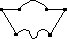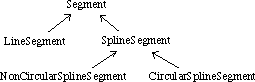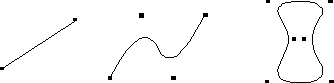## 4.1 SegmentsOne can think of a shape as the boundaries of the graphical object, where the boundaries are made of segments. Straight line boundaries are made of line segments and curved boundaries of spline segments. It is possible to combine both line and spline segments in the construction of a shape, as can be seen in in example in the margin using four line segments and two spline segments.

As can be seen in the Figure below, there are three kinds of segments: line, non-circular spline and circular spline segments.

Figure 5: The segment hierarchyA line segment is a straight line between two end points. A spline segment is spanned by at least three control points. There are two kinds of spline segments: an non-circular spline that terminates in its two extreme control points and a circular spline that do not touch any of its control points and does not have a start nor an ending point. Except for the two end points of a non-circular spline, the control points of a spline segment does not lie on the curve. Instead the control points are distant to the curve and act like 'magnets' pulling the curve. See the Figure below, which shows examples of the three segment types. The quadratic dots are the control points defining the segments.

Figure 6: Examples of SegmentsIt is possible to construct any kind of shape using the three segment types. Any kind of shape with non-curved sides such as triangles, rectangles and polygons with an unlimited number of edges can be constructed from line segments. Circles and ellipses can be represented with circular spline segments. Even objects consisting of a combination of spline and line segments can be constructed. Since shapes represent the outlines of graphical objects, it is possible to construct any kind of graphical object as long as the object has well defined boundaries.

 The Bifrost Graphics System - Reference Manual © 1991-2004 Mjølner Informatics [Modified: Tuesday October 24th 2000 at 15:02]